## Introduction¶

The goal of this notebook is to investigate and explore a method of time-stretching an existing audio track such that its rhythmic pulses become expanded or contracted along the Fibonacci sequence, using Euclidean rhythms as the basis for modification. For lack of a better term, let's call it Fibonacci stretch.

Inspiration for this came initially from Vijay Iyer's article on Fibonacci numbers and musical rhythm as well as his trio's renditions of "Mystic Brew" and "Human Nature"; we'll use original version of the latter as an example throughout. We'll also touch upon Godfried Toussaint's work on Euclidean rhythms and Bjorklund's algorithm, both of which are intimately related.

### A sneak peek at the final result¶

This is where we'll end up:

In :
import IPython.display as ipd
ipd.Audio("data/out_humannature_90s_stretched.mp3", rate=44100)

Out:

## Part 1 - Representing rhythm as symbolic data¶

### 1.1 Rhythms as arrays¶

The main musical element we're going to play with here is rhythm (in particular, rhythmic ratios and their similarities). The base rhythm that we're going to focus on is the tresillo ("three"-side) of the son clave pattern, which sounds like this:

In :
ipd.Audio("data/tresillo_rhythm.mp3", rate=44100)

Out:

...and looks something like this in Western music notation: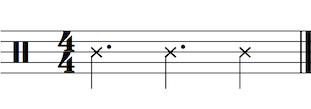We can convert that into a sequence of bits, with each 1 representing an onset, and 0 representing a rest (similar to the way a sequencer works). Doing so yields this:

[1 0 0 1 0 0 1 0]

...which we can conveniently store as a list in Python. Actually, this is a good time to start diving directly into code. First, let's import all the Python libraries we need:

In :
%matplotlib inline
import math # Standard library imports
import IPython.display as ipd, librosa, librosa.display, numpy as np, matplotlib.pyplot as plt # External libraries
import bjorklund # Fork of Brian House's implementation of Bjorklund's algorithm https://github.com/brianhouse/bjorklund


Briefly: we're using IPython.display to do audio playback, librosa for the bulk of the audio processing and manipulation (namely time-stretching), numpy to represent data, and matplotlib to plot the data.

Here's our list of bits encoding the tresillo sequence in Python (we'll use numpy arrays for consistency with later when when we deal with both audio signals and plotting visualizations):

In :
tresillo_rhythm = np.array([1, 0, 0, 1, 0, 0, 1, 0])
print(tresillo_rhythm)

[1 0 0 1 0 0 1 0]


Note that both the music notation and the array are symbolic representations of the rhythm; the rhythm is abstracted so that there is no information about tempo, dynamics, timbre, or other musical information. All we have is the temporal relationship between each note in the sequence (as well as the base assumption that the notes are evenly spaced).

Let's hear (and visualize) an example of how this rhythm sounds in more concrete terms:

In :
# Generate tresillo clicks
sr = 44100
tresillo_click_interval = 0.25 # in seconds
tresillo_click_times = np.array([i * tresillo_click_interval for i in range(len(tresillo_rhythm))
if tresillo_rhythm[i] != 0])
tresillo_clicks = librosa.clicks(times=tresillo_click_times, click_freq=2000.0, sr=sr) # Generate clicks according to the rhythm

# Plot clicks and click times
plt.figure(figsize=(8, 2))
librosa.display.waveplot(tresillo_clicks, sr=sr)
plt.vlines(tresillo_click_times + 0.005, -1, 1, color="r") # Add tiny offset so the first line shows up
plt.xticks(np.arange(0, 1.75, 0.25))

# Render clicks as audio
ipd.Audio(tresillo_clicks, rate=sr)

Out: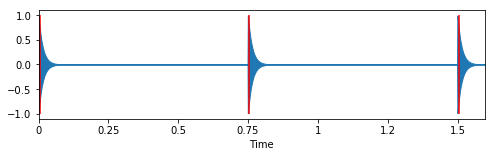### 1.2 Rhythmic properties¶

To work with rhythm in an analytical fashion, we'll need to define some properties of a given rhythmic sequence.

Let's define pulses as the number of onsets in a sequence (i.e. the number of 1s as opposed to 0s), and steps as the total number of elements in the sequence:

In :
tresillo_num_pulses = np.count_nonzero(tresillo_rhythm)
tresillo_num_steps = len(tresillo_rhythm)
print("The tresillo rhythm has {} pulses and {} steps".format(tresillo_num_pulses, tresillo_num_steps))

The tresillo rhythm has 3 pulses and 8 steps


We can listen to the pulses and steps together:

In :
def generate_rhythm_clicks(rhythm, click_interval=0.25, sr=44100):
step_length_samples = int(librosa.time_to_samples(click_interval, sr=sr))
rhythm_length_samples = step_length_samples * (len(rhythm))

# Generate click times
pulse_click_times, step_click_times = generate_rhythm_times(rhythm, click_interval)

# Generate pulse clicks
pulse_click_times = np.array([i * click_interval for i in range(len(rhythm))
if rhythm[i] != 0])
pulse_clicks = librosa.clicks(times=pulse_click_times, click_freq=2000.0, sr=sr, length=rhythm_length_samples)

# Generate step clicks
step_click_times = np.array([i * click_interval for i in range(len(rhythm))])
step_clicks = librosa.clicks(times=step_click_times, click_freq=1000.0, sr=sr, length=rhythm_length_samples)
step_clicks = np.hstack((step_clicks, np.zeros(step_length_samples, dtype="int"))) # add last step samples

# Add zeros to pulse clicks so that it's the same length as the step clicks signal
pulse_clicks = np.hstack((pulse_clicks, np.zeros(len(step_clicks)-len(pulse_clicks), dtype="int")))

# Ensure proper length
pulse_clicks = pulse_clicks[:rhythm_length_samples]
step_clicks = step_clicks[:rhythm_length_samples]

return (pulse_clicks, step_clicks)

def generate_rhythm_times(rhythm, interval):
pulse_times = np.array([float(i * interval) for i in range(len(rhythm)) if rhythm[i] != 0])
step_times = np.array([float(i * interval) for i in range(len(rhythm))])
return (pulse_times, step_times)

# Generate the clicks
tresillo_pulse_clicks, tresillo_step_clicks = generate_rhythm_clicks(tresillo_rhythm, tresillo_click_interval)
tresillo_pulse_times, tresillo_step_times = generate_rhythm_times(tresillo_rhythm, tresillo_click_interval)

# Tresillo as an array
print(tresillo_rhythm)

# Tresillo audio, plotted
plt.figure(figsize=(8, 2))
librosa.display.waveplot(tresillo_pulse_clicks + tresillo_step_clicks, sr=sr)
plt.vlines(tresillo_pulse_times + 0.005, -1, 1, color="r")
plt.vlines(tresillo_step_times + 0.005, -0.5, 0.5, color="r")

# Tresillo as audio
ipd.Audio(tresillo_pulse_clicks + tresillo_step_clicks, rate=44100)

[1 0 0 1 0 0 1 0]

Out: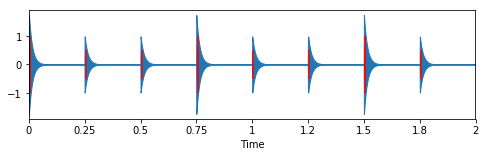You can follow along with the printed array and hear that every 1 corresponds to a pulse, and every 0 to a step.

In addition, let's define pulse lengths as the number of steps that each pulse lasts:

In :
# Function to calculate pulse lengths based on rhythm patterns
def calculate_pulse_lengths(rhythm):
pulse_lengths = np.array(([i for i,p in enumerate(rhythm) if p > 0]))
pulse_lengths = np.hstack((pulse_lengths, len(rhythm)))
pulse_lengths = np.array([pulse_lengths[i+1] - pulse_lengths[i] for i in range(len(pulse_lengths) - 1)])

return pulse_lengths

tresillo_pulse_lengths = calculate_pulse_lengths(tresillo_rhythm)
print("Tresillo pulse lengths: {}".format(tresillo_pulse_lengths))

Tresillo pulse lengths: [3 3 2]


Note that the tresillo rhythm's pulse lengths all fall along the Fibonacci sequence. This allows us do some pretty fun things, as we'll see in a bit. But first let's take a step back.

## Part 2 - Fibonacci rhythms¶

### 2.1 Fibonacci numbers¶

The Fibonacci sequence is a particular sequence in which each value is the sum of the two preceding values. We can define a function in Python that gives us the nth Fibonacci number:

In :
def fibonacci(n):
if n == 0 or n == 1:
return n
else:
return fibonacci(n-1) + fibonacci(n-2)


And the first 20 numbers in the sequence are:

In :
first_twenty_fibs = np.array([fibonacci(n) for n in range(20)])
plt.figure(figsize=(16,1))
plt.scatter(first_twenty_fibs, np.zeros(20), c="r")
plt.axis("off")
print(first_twenty_fibs)

[   0    1    1    2    3    5    8   13   21   34   55   89  144  233  377
610  987 1597 2584 4181]The Fibonacci sequence is closely linked to the golden ratio in many ways, including the fact that as we go up the sequence, the ratio between successive numbers gets closer and closer to the golden ratio. (If you're interested, Vijay Iyer's article Strength in numbers: How Fibonacci taught us how to swing goes into this in more depth.)

Below is a plot of Fibonacci number ratios in red, and the golden ratio as a constant in blue. You can see how the Fibonacci ratios converge to the golden ratio:

In :
# Calculate and plot Fibonacci number ratios
phi = (1 + math.sqrt(5)) / 2 # Golden ratio; 1.61803398875...
fibs_ratios = np.array([first_twenty_fibs[i] / float(max(1, first_twenty_fibs[i-1])) for i in range(2,20)])
plt.plot(np.arange(len(fibs_ratios)), fibs_ratios, "r")

# Plot golden ratio as a consant
phis = np.empty(len(fibs_ratios))
phis.fill(phi)
plt.xticks(np.arange(len(fibs_ratios)))
plt.xlabel("Fibonacci index (denotes i for ith Fibonacci number)")
plt.ylabel("Ratio between ith and (i-1)th Fibonacci number")
plt.plot(np.arange(len(phis)), phis, "b", alpha=0.5)

Out:
[<matplotlib.lines.Line2D at 0x10c132b90>]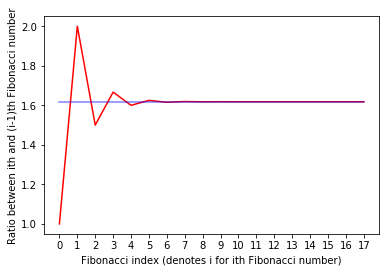We can also use the golden ratio to find the index of a Fibonacci number:

In :
def find_fibonacci_index(n):
phi = (1 + math.sqrt(5)) / 2 # Golden ratio; 1.61803398875...
return int(math.log((n * math.sqrt(5)) + 0.5) / math.log(phi))

fib_n = 21
fib_i = find_fibonacci_index(fib_n)
assert(fibonacci(fib_i) == fib_n)
print("{} is the {}th Fibonacci number".format(fib_n, fib_i))

21 is the 8th Fibonacci number


### 2.2 Using Fibonacci numbers to manipulate rhythms¶

Recall our tresillo rhythm:

In :
plt.figure(figsize=(8, 2))
plt.vlines(tresillo_pulse_times + 0.005, -1, 1, color="r")
plt.vlines(tresillo_step_times + 0.005, -0.5, 0.5, color="r", alpha=0.5)
plt.yticks([])

print("Tresillo rhythm sequence: {}".format(tresillo_rhythm))
print("Tresillo pulse lengths: {}".format(tresillo_pulse_lengths))

Tresillo rhythm sequence: [1 0 0 1 0 0 1 0]
Tresillo pulse lengths: [3 3 2]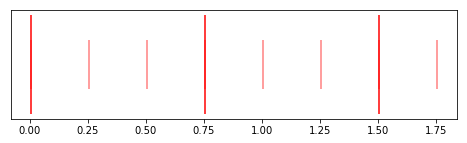We might classify it as a Fibonacci rhythm, since every one of its pulse lengths is a Fibonacci number. If we wanted to expand that rhythm along the Fibonacci sequence, what would that look like?

An intuitive (and, as it turns out, musically satisfying) method would be to take every pulse length and simply replace it with the Fibonacci number that follows it. So in our example, the 3s become 5s, and the 2 becomes 3.

In :
def fibonacci_expand_pulse_lengths(pulse_lengths):
new_pulse_lengths = np.array([], dtype="int")
for pulse_length in pulse_lengths:
fib_i = find_fibonacci_index(pulse_length)
new_pulse_length = fibonacci(fib_i + 1)
new_pulse_lengths = np.hstack((new_pulse_lengths, new_pulse_length))
return new_pulse_lengths

print("Expanded tresillo pulse lengths: {}".format(fibonacci_expand_pulse_lengths(tresillo_pulse_lengths)))

Expanded tresillo pulse lengths: [5 5 3]


We'll also want to be able to contract rhythms along the Fibonacci sequence (i.e. choose numbers in decreasing order instead of increasing order), as well as specify how many Fibonacci numbers away we want to end up.

We can generalize this expansion and contraction into a single function that can scale pulse lengths:

In :
# Function to scale pulse lengths along the Fibonacci sequence
#
# Note that scale_amount determines the direction and magnitude of the scaling.
# If scale_amount > 0, it corresponds to a rhythmic expansion.
# If scale_amount < 0, it corresponds to a rhythmic contraction.
# If scale_amount == 0, the original scale is maintained and no changes are made.
def fibonacci_scale_pulse_lengths(pulse_lengths, scale_amount=0):
scaled_pulse_lengths = np.array([], dtype="int")
for pulse_length in pulse_lengths:
fib_i = find_fibonacci_index(pulse_length)
# if fib_i + scale_amount < 0:
#     print("ERROR: Scale amount out of bounds")
#     return pulse_lengths
scaled_pulse_length = fibonacci(max(fib_i + scale_amount, 0))
scaled_pulse_lengths = np.hstack((scaled_pulse_lengths, scaled_pulse_length))
return scaled_pulse_lengths

print("Tresillo pulse lengths:                 {}".format(tresillo_pulse_lengths))
print("Tresillo pulse lengths expanded by 1:   {}".format(fibonacci_scale_pulse_lengths(tresillo_pulse_lengths, 1)))
print("Tresillo pulse lengths expanded by 2:   {}".format(fibonacci_scale_pulse_lengths(tresillo_pulse_lengths, 2)))
print("Tresillo pulse lengths contracted by 1: {}".format(fibonacci_scale_pulse_lengths(tresillo_pulse_lengths, -1)))

Tresillo pulse lengths:                 [3 3 2]
Tresillo pulse lengths expanded by 1:   [5 5 3]
Tresillo pulse lengths expanded by 2:   [8 8 5]
Tresillo pulse lengths contracted by 1: [2 2 1]


Of course, once we have these scaled pulse lengths, we'll want to be able to convert them back into rhythms, in our original array format:

In :
# Define the functions we'll use to scale rhythms along the Fibonacci sequence
def fibonacci_scale_rhythm(rhythm, scale_amount):
pulse_lengths = calculate_pulse_lengths(rhythm)
scaled_pulse_lengths = fibonacci_scale_pulse_lengths(pulse_lengths, scale_amount)
scaled_pulse_lengths = np.array([p for p in scaled_pulse_lengths if p > 0])

scaled_rhythm = pulse_lengths_to_rhythm(scaled_pulse_lengths)
return scaled_rhythm

def pulse_lengths_to_rhythm(pulse_lengths):
rhythm = np.array([], dtype="int")
for p in pulse_lengths:
pulse = np.zeros(p, dtype="int")
pulse = 1
rhythm = np.hstack((rhythm, pulse))
return rhythm

# Scale tresillo rhythm by a variety of factors and plot the results
for scale_factor, color in [(0, "r"), (1, "g"), (2, "b"), (-1, "y")]:
scaled_rhythm = fibonacci_scale_rhythm(tresillo_rhythm, scale_factor)
scaled_pulse_indices = np.array([p_i for p_i,x in enumerate(scaled_rhythm) if x > 0 ])
scaled_step_indices = np.array([s_i for s_i in range(len(scaled_rhythm))])
scaled_pulse_ys = np.empty(len(scaled_pulse_indices))
scaled_pulse_ys.fill(0)
scaled_step_ys = np.empty(len(scaled_step_indices))
scaled_step_ys.fill(0)

# plt.figure(figsize=(len([scaled_rhythm])*0.5, 1))
plt.figure(figsize=(8, 1))
if scale_factor > 0:
plt.title("Tresillo rhythm expanded by {}: {}".format(abs(scale_factor), scaled_rhythm), loc="left")
elif scale_factor < 0:
plt.title("Tresillo rhythm contracted by {}: {}".format(abs(scale_factor), scaled_rhythm), loc="left")
else: # scale_factor == 0, which means rhythm is unaltered
plt.title("Tresillo rhythm: {}".format(scaled_rhythm), loc="left")
# plt.scatter(scaled_pulse_indices, scaled_pulse_ys, c=color)
# plt.scatter(scaled_step_indices, scaled_step_ys, c="k", alpha=0.5)
# plt.grid(True)
plt.vlines(scaled_pulse_indices, -1, 1, color=color)
plt.vlines(scaled_step_indices, -0.5, 0.5, color=color, alpha=0.5)
plt.xticks(np.arange(0, plt.xlim(), 1))
plt.yticks([])
# plt.xticks(np.linspace(0, 10, 41))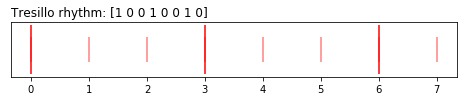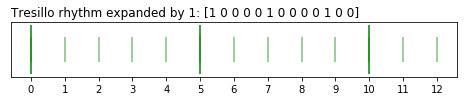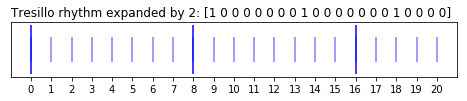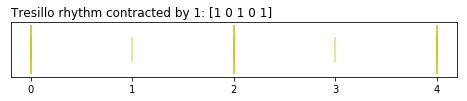This is exactly the kind of rhythmic expansion and contraction that the Vijay Iyer Trio explore in their renditions of "Mystic Brew" and "Human Nature (Trio Extension)".

Next up, let's begin working with some actual audio!

## Part 3 - Mapping rhythm to audio¶

Part of the beauty of working with rhythms in a symbolic fashion is that once we set things up, we can apply them to any existing audio track.

To properly map the relationship between a rhythmic sequence and an audio representation of a piece of music, we'll have to do some feature extraction, that is, teasing out specific attributes of the music by analyzing the audio signal.

Our goal is to create a musically meaningful relationship between our symbolic rhythmic data and the audio track we want to manipulate.

### 3.1 Estimating tempo¶

First we'll load up our source audio file. For this example we'll work with Michael Jackson's "Human Nature", off of his 1982 album Thriller:

In :
# Load input audio file
filename = "data/humannature_30s.mp3"

plt.figure(figsize=(16,4))
librosa.display.waveplot(y, sr=sr)

ipd.Audio(y, rate=sr)

Out: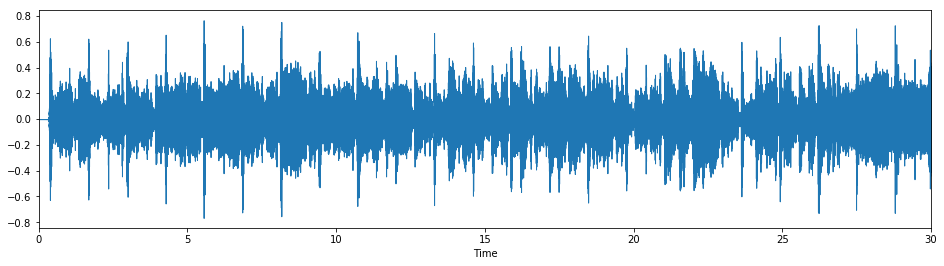An important feature we want to extract from the audio is tempo (i.e. the time interval between steps). Let's estimate that using the librosa.beat.tempo method (which requires us to first detect onsets, or []):

In :
def estimate_tempo(y, sr, start_bpm=120.0):
# Estimate tempo
onset_env = librosa.onset.onset_strength(y, sr=sr) # TODO: Compare this with librosa.beat.beat_track
tempo = librosa.beat.tempo(y, sr=sr, onset_envelope=onset_env, start_bpm=start_bpm)

return float(tempo)

tempo = estimate_tempo(y, sr)
print("Tempo (calculated): {}".format(tempo))
tempo = 93.0 # Hard-coded from prior knowledge
print("Tempo (hard-coded): {}".format(tempo))

Tempo (calculated): 92.28515625
Tempo (hard-coded): 93.0

(We can see that the tempo we've estimated differs by approximately 1BPM from the tempo that we've hard-coded from prior knowledge. It's often the case that such automatic feature extraction tools and algorithms require a fair bit of fine-tuning, so we can improve our results by supplying some user-defined parameters, especially when using them out of the box like we are here. The variables hop_length and tempo are two such parameters in this case. However, the more parameters we define manually, the less flexible our overall system becomes, so it's a tradeoff between accuracy and robustness.)

### 3.2 From tempo to beats¶

From the tempo we can calculate the times of every beat in the song (assuming the tempo is consistent, which in this case it is):

In :
# Calculate beat times
def calculate_beat_times(y, sr, tempo):
# Calculate params based on input
T = len(y)/float(sr) # Total audio length in seconds
seconds_per_beat = 60.0/tempo

# Start beat at first onset rather than time 0
# TODO: Let this first onset also be user-supplied for more accurate results
beat_times = np.arange(detect_first_onset_time(y, sr), T, seconds_per_beat)

return beat_times

# Detect first onset
def detect_first_onset_time(y, sr, hop_length=1024):
onset_frames = librosa.onset.onset_detect(y, sr=sr, hop_length=hop_length)
onset_times = librosa.frames_to_time(onset_frames)
return onset_times

beat_times = calculate_beat_times(y, sr, tempo)
print("First 10 beat times (in seconds): {}".format(beat_times[:10]))

First 10 beat times (in seconds): [ 0.37151927  1.01668056  1.66184186  2.30700315  2.95216444  3.59732573
4.24248702  4.88764831  5.5328096   6.17797089]


And let's listen to our extracted beats with the original audio track:

In :
# Listen to beat clicks (i.e. a metronome)
beat_clicks = librosa.clicks(times=beat_times, sr=sr, length=len(y))

# Plot waveform and beats
plt.figure(figsize=(16,4))
librosa.display.waveplot(y, sr=sr)
plt.vlines(beat_times, -0.25, 0.25, color="r")

ipd.Audio(y + beat_clicks, rate=sr)

Out: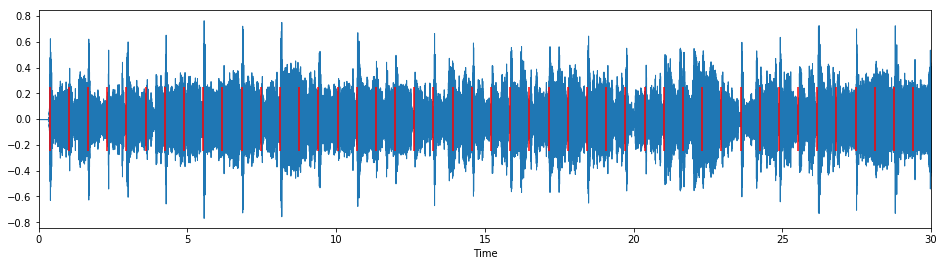### 3.3 From beats to measures¶

In order to map our tresillo rhythm to the audio in a musically meaningful way, we'll need to group beats into measures. From listening to the above example we can hear that every beat corresponds to a quarter note; thus, we'll set beats_per_measure to 4:

In :
beats_per_measure = 4


Using beats_per_measure we can calculate the times for the start of each measure:

In :
# Calculate measure indices in samples
def calculate_measure_samples(y, beat_samples, beats_per_measure):
max_samples = len(y)
start_sample = beat_samples
beat_interval = beat_samples - beat_samples
measure_interval = beat_interval * beats_per_measure
if measure_interval >= beat_interval:
return np.array(beat_samples[::beats_per_measure], dtype="int")
else:
beat_indices = np.indices([len(beat_samples)])
measure_indices = np.indices([len(beat_samples)/beats_per_measure])
return np.interp(measure_indices, beat_indices/beats_per_measure, beat_samples)

# Work in samples from here on
beat_samples = librosa.time_to_samples(beat_times, sr=sr)
measure_samples = calculate_measure_samples(y, beat_samples, beats_per_measure)

print("First 10 measure samples: {}".format(measure_samples[:10]))

First 10 measure samples: [  16384  130190  243996  357803  471609  585416  699222  813029  926835
1040642]


Note that we're working in samples now, as this is the unit that the audio data is actually stored in; when we loaded up the audio track, we essentially read in a large array of samples. The sample rate, which we defined as sr, tells us how many samples there are per second.

Thus, it's a simple matter to convert samples to times whenever we need to:

In :
measure_times = librosa.samples_to_time(measure_samples, sr=sr)
print("First 10 measure times (in seconds): {}".format(measure_times[:10], sr=sr))

First 10 measure times (in seconds): [  0.37151927   2.9521542    5.53278912   8.11344671  10.69408163
13.27473923  15.85537415  18.43603175  21.01666667  23.59732426]


We can visualize, and listen to, the measure and beat markers along with the original waveform:

In :
# Add clicks, then plot and listen
plt.figure(figsize=(16, 4))
librosa.display.waveplot(y, sr=sr)

plt.vlines(measure_times, -1, 1, color="r")
plt.vlines(beat_times, -0.5, 0.5, color="r")

measure_clicks = librosa.clicks(times=measure_times, sr=sr, click_freq=3000.0, length=len(y))
ipd.Audio(y + measure_clicks + beat_clicks, rate=sr)

Out: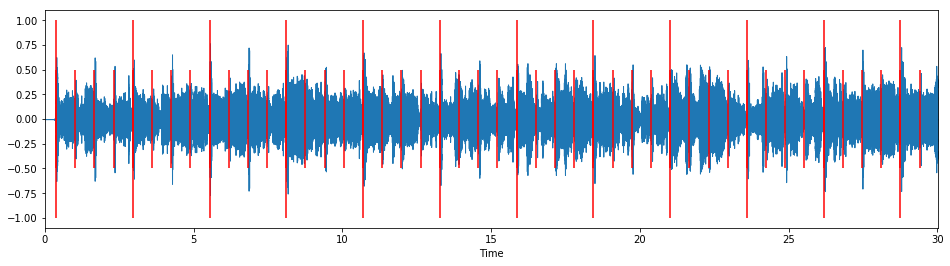### 3.4 Putting it all together: mapping symbolic rhythms to audio signals¶

With our knowledge of the song's tempo, beats, and measures, we can start bringing our symbolic rhythms into audio-land. Again, let's work with our trusty tresillo rhythm:

In :
print("Tresillo rhythm: {}\n"
"{} pulses, {} steps".format(tresillo_rhythm, tresillo_num_pulses, tresillo_num_steps))

Tresillo rhythm: [1 0 0 1 0 0 1 0]
3 pulses, 8 steps


For this example, we want the rhythm to last an entire measure as well, so we'll set steps_per_measure to be the number of steps in the rhythm (in this case, 8):

In :
steps_per_measure = tresillo_num_steps
steps_per_measure

Out:
8

With these markers in place, we can now overlay the tresillo rhythm onto each measure and listen to the result:

In :
"""Generating clicks for tresillo rhythm at the proper tempo and start time,
to overlay onto an audio track"""
def generate_rhythm_overlay(rhythm, measure_samples, steps_per_measure):
# Calculate click interval
measure_length = measure_samples-measure_samples
# click_tempo = tempo * (steps_per_measure/float(beats_per_measure))
# click_interval = 60.0/click_tempo
measure_length_seconds = librosa.samples_to_time(measure_length, sr=sr)
click_interval = measure_length_seconds / float(steps_per_measure)

# Generate click times for single measure
pulse_times_measure, step_times_measure = generate_rhythm_times(rhythm, click_interval)

# Generate clicks for single measure
pulse_clicks_measure, step_clicks_measure = generate_rhythm_clicks(rhythm, click_interval, sr=sr)

# Concatenate clicks and click times for all measures
pulse_times, step_times, pulse_clicks, step_clicks = np.array([]), np.array([]), np.array([]), np.array([])
for s in measure_samples:
t = float(librosa.samples_to_time(s, sr=sr))
pulse_clicks = np.hstack((pulse_clicks, pulse_clicks_measure))
step_clicks = np.hstack((step_clicks, step_clicks_measure))
pulse_times = np.hstack((pulse_times, pulse_times_measure + t))
step_times = np.hstack((step_times, step_times_measure + t))

# Offset clicks by first onset
pulse_clicks = np.hstack((np.zeros(measure_samples), pulse_clicks))
step_clicks = np.hstack((np.zeros(measure_samples), step_clicks))

return (pulse_times, step_times, pulse_clicks, step_clicks)

"""Visualizing and hearing the result"""
def overlay_rhythm_onto_audio(rhythm, audio_samples, measure_samples, sr=44100, click_colors={"measure": "r",
"pulse": "r",
"step": "r"}):

# Get overlay data
pulse_times, step_times, pulse_clicks, step_clicks = generate_rhythm_overlay(rhythm,
measure_samples,
len(rhythm))
measure_times = librosa.samples_to_time(measure_samples, sr=sr)
measure_clicks = librosa.clicks(times=measure_times, sr=sr, click_freq=3000.0, length=len(audio_samples))

# Calculate max length in samples
available_lengths = [len(audio_samples), len(measure_clicks), len(pulse_clicks), len(step_clicks)]
length_samples = min(available_lengths)

# Plot original waveform
plt.figure(figsize=(16, 4))
librosa.display.waveplot(audio_samples, sr=sr, alpha=0.5)

# Plot rhythm clicks
plt.vlines(measure_times, -1, 1, color=click_colors["measure"])
plt.vlines(pulse_times, -0.5, 0.5, color=click_colors["pulse"])
plt.vlines(step_times, -0.25, 0.25, color=click_colors["step"], alpha=0.75)

# Play both clicks together with audio track
concatenated_audio_samples = ((audio_samples[:length_samples]*2.0)
+ (measure_clicks[:length_samples]*0.25)
+ (pulse_clicks[:length_samples]*0.25)
+ (step_clicks[:length_samples]*0.25))
audio_display = ipd.Audio(concatenated_audio_samples, rate=sr)
return audio_display

overlay_rhythm_onto_audio(tresillo_rhythm, y, measure_samples, sr=sr)

Out: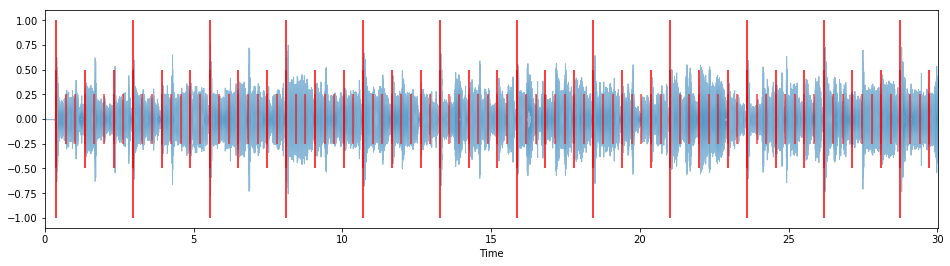The clicks for measures, pulses, and steps, overlap with each other at certain points. While you can hear this based on the fact that each click is at a different frequency, it can be hard to tell visually in the above figure. We can make this more apparent by plotting each set of clicks with a different color.

In the below figure, each measure is denoted by a large red line, each pulse by a medium green line, and each step by a small blue line.

In :
overlay_rhythm_onto_audio(tresillo_rhythm, y, measure_samples, sr=sr, click_colors={"measure": "r",
"pulse": "g",
"step": "b"})

Out: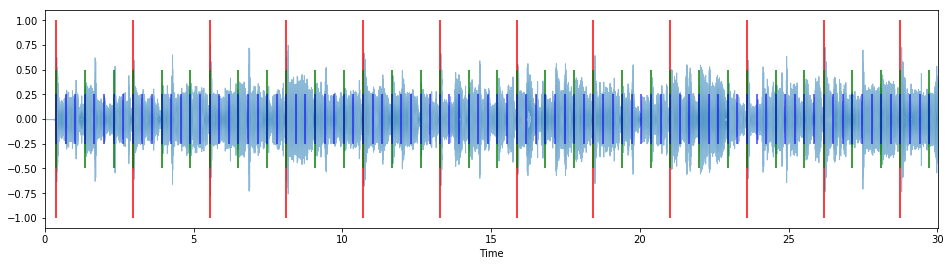You can hear that the tresillo rhythm's pulses line up with the harmonic rhythm of "Human Nature"; generally, we want to pick rhythms and audio tracks that have at least some kind of musical relationship.

(We could actually try to estimate rhythmic patterns based on onsets and tempo, but that's for another time.)

## Part 4 - Time-stretching audio¶

Now that we've put the symbolic rhythm and source audio together, we're ready to begin manipulating the audio and doing some actual stretching!

### 4.1 Target rhythms¶

First, we'll define the target rhythm that we want the audio to be mapped to:

In :
original_rhythm = tresillo_rhythm
target_rhythm = fibonacci_scale_rhythm(original_rhythm, 1) # "Fibonacci scale" original rhythm by a factor of 1
print("Original rhythm: {}\n"
"Target rhythm:   {}".format(original_rhythm, target_rhythm))

Original rhythm: [1 0 0 1 0 0 1 0]
Target rhythm:   [1 0 0 0 0 1 0 0 0 0 1 0 0]


### 4.2 Pulse ratios¶

Given an original rhythm and target rhythm, we can compute their pulse ratios, that is, the ratio between each of their pulses:

In :
# Calculate ratios between pulses for two rhythm sequences
# NOTE: This assumes that both rhythm sequences have the same number of pulses!
def calculate_pulse_ratios(original_rhythm, target_rhythm):
original_pulse_lengths = calculate_pulse_lengths(original_rhythm)
target_pulse_lengths = calculate_pulse_lengths(target_rhythm)
num_pulses = min(len(original_pulse_lengths), len(target_pulse_lengths))
pulse_ratios = np.array([original_pulse_lengths[i]/float(target_pulse_lengths[i]) for i in range(num_pulses)])
return pulse_ratios

print("Pulse ratios: {}".format(calculate_pulse_ratios(original_rhythm, target_rhythm)))

Pulse ratios: [ 0.6         0.6         0.66666667]


### 4.3 Modifying measures by time-stretching¶

Since we're treating our symbolic rhythms as having the duration of one measure, it makes sense to start by modifying a single measure.

Basically what we want to do is: for each pulse, get the audio chunk that maps to that pulse, and time-stretch it based on our calculated pulse ratios.

Below is an implementation of just that. It's a bit long, but that's mostly due to having to define several properties to do with rhythm and audio. The core idea, of individually stretching the pulses, remains the same:

In :
# Modify a single measure
def modify_measure(data, original_rhythm, target_rhythm, stretch_method):
modified_data = np.array([])

# Define the rhythmic properties we'll use
original_num_samples = len(data)
original_num_steps = len(original_rhythm)
target_num_steps = len(target_rhythm)

# Get indices of steps for measure
original_step_interval = original_num_samples / float(original_num_steps)
original_step_indices = np.arange(0, original_num_samples, original_step_interval, dtype="int")

# Get only indices of pulses based on rhythm
original_pulse_indices = np.array([original_step_indices[i] for i in range(original_num_steps) if original_rhythm[i] > 0])

# Calculate pulse ratios
pulse_ratios = calculate_pulse_ratios(original_rhythm, target_rhythm)

# Calculate pulse lengths
original_pulse_lengths = calculate_pulse_lengths(original_rhythm)
target_pulse_lengths = calculate_pulse_lengths(target_rhythm)

# Concatenate time-stretched versions of rhythm's pulses
for i,p in enumerate(original_pulse_indices):
# Get pulse sample data; samples between current and next pulse, or if it's the final pulse,
# samples between pulse and end of audio
pulse_start = p
pulse_stop = len(data)-1
if i < len(original_pulse_indices)-1:
pulse_stop = original_pulse_indices[i+1]
pulse_samples = data[pulse_start:pulse_stop]

# Time-stretch this step based on ratio of old to new rhythm length
# TODO: Try out other methods of manipulation, such as using onset detection in addition to steps and pulses
if stretch_method == "timestretch":
pulse_samples = librosa.effects.time_stretch(pulse_samples, pulse_ratios[i])
elif stretch_method == "euclidean":
pulse_samples = euclidean_stretch(pulse_samples,
original_pulse_lengths[i],
target_pulse_lengths[min(i, len(target_pulse_lengths)-1)])
else:
print("ERROR: Invalid stretch method {}".format(stretch_method))

# Add the samples to our modified audio time series
modified_data = np.hstack((modified_data, pulse_samples))

# Time-stretch entire measure to maintain original measure length (so that it sounds more natural)
stretch_multiplier = len(modified_data)/float(len(data))
modified_data = librosa.effects.time_stretch(modified_data, stretch_multiplier)

return modified_data


You'll notice that in the part where we choose stretch methods, there's a function called euclidean_stretch that we haven't defined. We'll get to that in just a second! For now, let's just put a stub there:

In :
# Euclidean stretch STUB
def euclidean_stretch(pulse_samples, original_pulse_length, target_pulse_length):
return pulse_samples


... so that we can hear what our modification method sounds like when applied to the first measure of "Human Nature":

In :
first_measure_data = y[measure_samples:measure_samples]
first_measure_modified = modify_measure(first_measure_data,
original_rhythm, target_rhythm,
stretch_method="timestretch")
ipd.Audio(first_measure_modified, rate=sr)

Out:

It doesn't sound like there's much difference between the stretched version and the original, does it?

### 4.4 Modifying an entire track by naively time-stretching each pulse¶

To get a better sense, let's apply the modification to the entire audio track:

In :
# Modify an entire audio track; basically just loops through a track's measures
# and calls modify_measure() on each measure
def modify_track(data, measure_samples, original_rhythm, target_rhythm, stretch_method="timestretch"):
modified_track_data = np.zeros(measure_samples)
modified_measure_samples = np.array([], dtype="int")
for i, sample in enumerate(measure_samples[:-1]):
modified_measure_samples = np.hstack((modified_measure_samples, len(modified_track_data)))
measure_start = measure_samples[i]
measure_stop = measure_samples[i+1]
measure_data = data[measure_start:measure_stop]
modified_measure_data = modify_measure(measure_data, original_rhythm, target_rhythm, stretch_method)
modified_track_data = np.hstack((modified_track_data, modified_measure_data))
return (modified_track_data, modified_measure_samples)

# Modify the track using naive time-stretch
y_modified, measure_samples_modified = modify_track(y, measure_samples,
original_rhythm, target_rhythm,
stretch_method="timestretch")

plt.figure(figsize=(16,4))
librosa.display.waveplot(y_modified, sr=sr)
ipd.Audio(y_modified, rate=sr)

Out: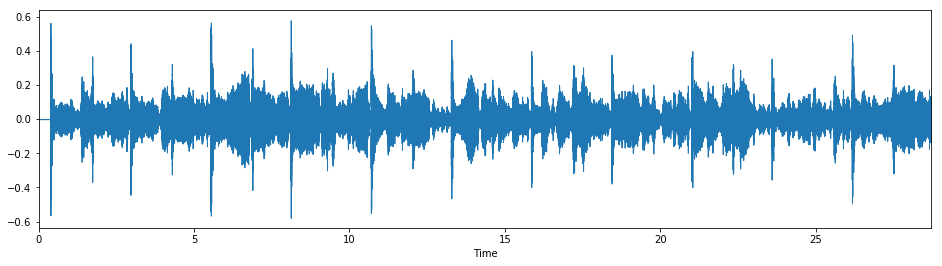Listening to the whole track, only perceptible difference is that the last two beats of each measure are slightly faster. If we look at the pulse ratios again:

In :
print(calculate_pulse_ratios(original_rhythm, target_rhythm))

[ 0.6         0.6         0.66666667]


... we can see that this makes sense, as we're time-stretching the first two pulses by the same amount, and then time-stretching the last pulse by a different amount.

(Note that while we're expanding our original rhythm along the Fibonacci sequence, this actually corresponds to a contraction when time-stretching. This is because we want to maintain the original tempo, so we're trying to fit more steps into the same timespan.)

### 4.5 Overlaying target rhythm clicks¶

We can get some more insight if we sonify the target rhythm's clicks and overlay it onto our modified track:

In :
overlay_rhythm_onto_audio(target_rhythm, y_modified, measure_samples, sr)

Out: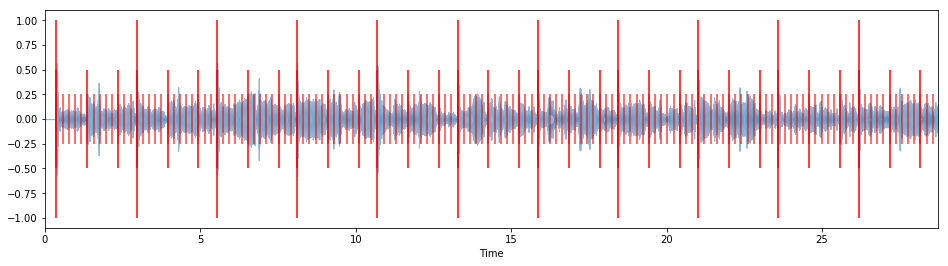This gets to the heart of the problem: when we time-stretch an entire pulse this way, we retain the original pulse's internal rhythm, essentially creating a polyrhythm in the target pulse's step (i.e. metrical) structure. Even though we're time-stretching each pulse, we don't hear a difference because everything within the pulse gets time-stretched by the same amount.

## Part 5 - Euclidean stretch¶

Listening to the rendered track in Part 4.5, you can hear that aside from the beginning of each measure and pulse, the musical onsets in the modified track don't really line up with the target rhythm's clicks at all. Thus, without the clicks, we have no way to identify the target rhythm, even though that's what we were using as the basis of our stretch method!

So how do we remedy this?

### 5.1 Subdividing pulses¶

We dig deeper. That is, we can treat each pulse as a rhythm of its own, and subdivide it accordingly, since each pulse is comprised of multiple steps after all.

In :
print("Original rhythm: {}\n"
"Target rhythm:   {}".format(original_rhythm, target_rhythm))

Original rhythm: [1 0 0 1 0 0 1 0]
Target rhythm:   [1 0 0 0 0 1 0 0 0 0 1 0 0]


Looking at the first pulses of the original rhythm and target rhythm, we want to turn

[1 0 0]

into

[1 0 0 0 0].

To accomplish this, we'll turn to the concept of Euclidean rhythms.

### 5.2 Generating Euclidean rhythms using Bjorklund's algorithm¶

A Euclidean rhythm is a type of rhythm that can be generated based upon the Euclidean algorithm for calculating the greatest common divisor of two numbers.

In :
def euclid(a, b):
m = max(a, b)
k = min(a, b)

if k==0:
return m
else:
return euclid(k, m%k)

print("Greatest common divisor of 8 and 12 is {}".format(euclid(8, 12)))

Greatest common divisor of 8 and 12 is 4


The concept of Euclidean rhythms was first introduced by Godfried Toussaint in his 2004 paper The Euclidean Algorithm Generates Traditional Musical Rhythms.

The algorithm for generating these rhythms is actually Bjorklund's algorithm, first described by E. Bjorklund in his 2003 paper The Theory of Rep-Rate Pattern Generation in the SNS Timing System, which deals with neutron accelerators in nuclear physics. Here we use Brian House's Python implementation of Bjorklund's algorithm; you can find the source code on GitHub.

It turns out that our tresillo rhythm is an example of a Euclidean rhythm. We can generate it by plugging in the number of pulses and steps into Bjorklund's algorithm:

In :
print(np.array(bjorklund.bjorklund(pulses=3, steps=8)))

[1 0 0 1 0 0 1 0]


### 5.3 Using Euclidean rhythms to subdivide pulses¶

Say we want to stretch a pulse [1 0 0] so that it resembles another pulse [1 0 0 0 0]:

In :
original_pulse = np.array([1,0,0])
target_pulse = np.array([1,0,0,0,0])


We want to know how much to stretch each subdivision. To do this, we'll convert these single pulses into rhythms of their own. First, we'll treat each step in the original pulse as an onset:

In :
original_pulse_rhythm = np.ones(len(original_pulse), dtype="int")
print(original_pulse_rhythm)

[1 1 1]


And as mentioned before, we'll use Bjorklund's algorithm to generate the target pulse's rhythm. The trick here is to use the number of steps in the original pulse as the number of pulses for the target pulse rhythm (hence the conversion to onsets earlier):

In :
target_pulse_rhythm = np.array(bjorklund.bjorklund(pulses=len(original_pulse), steps=len(target_pulse)))
print(target_pulse_rhythm)

[1 0 1 0 1]


You might have noticed that this rhythm is exactly the same as the rhythm produced by contracting the tresillo rhythm along the Fibonacci sequence by a factor of 1:

In :
print(fibonacci_scale_rhythm(tresillo_rhythm, -1))

[1 0 1 0 1]


And it's true that there is some significant overlap between Euclidean rhythms and Fibonacci rhythms. The advantage of working with Euclidean rhythms here is that they work with any number of pulses and steps, not just ones that are Fibonacci numbers.

To summarize:

In :
print("In order to stretch pulse-to-pulse {} --> {}\n"
"we subdivide and stretch rhythms   {} --> {}".format(original_pulse, target_pulse,
original_pulse_rhythm, target_pulse_rhythm))

In order to stretch pulse-to-pulse [1 0 0] --> [1 0 0 0 0]
we subdivide and stretch rhythms   [1 1 1] --> [1 0 1 0 1]


The resulting pulse ratios are:

In :
print(calculate_pulse_ratios(original_pulse_rhythm, target_pulse_rhythm))

[ 0.5  0.5  1. ]


... which doesn't intuitively look like it would produce something any different from what we tried before. However, we might perceive a greater difference because:

a) we're working on a more granular temporal level (subdivisions of pulses as opposed to measures), and

b) we're adjusting an equally-spaced rhythm (e.g. [1 1 1]) to one that's not necessarily equally-spaced (e.g. [1 0 1 0 1])

### 5.4 The Euclidean stretch algorithm¶

With all this in mind, we can now implement Euclidean stretch:

In :
# Euclidean stretch for modifying a single pulse (basically time-stretching subdivisions based on Euclidean rhythms)
def euclidean_stretch(pulse_samples, original_pulse_length, target_pulse_length):
target_pulse_samples = np.array([])

# Return empty samples array if target pulse length < 1
if target_pulse_length < 1:
return target_pulse_samples

# Ensure original pulse rhythm ("opr") has length equal to or less than target_pulse_length

# ... by using target pulse length
# original_pulse_length = min(original_pulse_length, target_pulse_length)

# ... by using divisors of original pulse length
# if original_pulse_length > target_pulse_length:
#     # print("WARNING: original_pulse_length {} "
#     #       "is greater than target_pulse_length {}".format(original_pulse_length,
#     #                                                       target_pulse_length))
#     for i in range(1, original_pulse_length+1):
#         opl_new = int(original_pulse_length / float(i))
#         if opl_new <= target_pulse_length:
#             original_pulse_length = opl_new
#             # print("original_pulse_length is now {}".format(original_pulse_length))
#             break

# ... by using lowest common multiple as target pulse length
if original_pulse_length > target_pulse_length:
# print("Target pulse length before: {}".format(target_pulse_length))
gcd = euclid(original_pulse_length, target_pulse_length)
lcm = (original_pulse_length*target_pulse_length) / gcd
target_pulse_length = lcm
# print("Target pulse length after: {}".format(target_pulse_length))
# original_pulse_length = target_pulse_length

opr = np.ones(original_pulse_length, dtype="int")

# Generate target pulse rhythm ("tpr")
tpr = bjorklund.bjorklund(pulses=original_pulse_length, steps=target_pulse_length)
tpr_pulse_lengths = calculate_pulse_lengths(tpr)
tpr_pulse_ratios = calculate_pulse_ratios(opr, tpr)

# Subdivide (i.e. segment) the pulse based on original pulse length
pulse_subdivision_step = int(len(pulse_samples) / float(original_pulse_length))
pulse_subdivision_indices = np.arange(0, len(pulse_samples), pulse_subdivision_step, dtype="int")
pulse_subdivision_indices = pulse_subdivision_indices[:original_pulse_length]

# Time-stretch each subdivision based on ratios
for i,si in enumerate(pulse_subdivision_indices):
subdivision_start = si
subdivision_stop = len(pulse_samples) - 1
if i < len(pulse_subdivision_indices)-1:
subdivision_stop = pulse_subdivision_indices[i+1]
pulse_subdivision_samples = pulse_samples[subdivision_start:subdivision_stop]

# Stretch the relevant subdivisions based on target pulse rhythm
pulse_subdivision_samples = librosa.effects.time_stretch(pulse_subdivision_samples, tpr_pulse_ratios[i])

# Concatenate phrase
target_pulse_samples = np.hstack((target_pulse_samples, pulse_subdivision_samples))

return target_pulse_samples


Let's take a listen to how it sounds:

In :
# Modify the track
y_modified, measure_samples_modified = modify_track(y, measure_samples,
original_rhythm, target_rhythm,
stretch_method="euclidean")

plt.figure(figsize=(16,4))
librosa.display.waveplot(y_modified, sr=sr)
ipd.Audio(y_modified, rate=sr)

Out: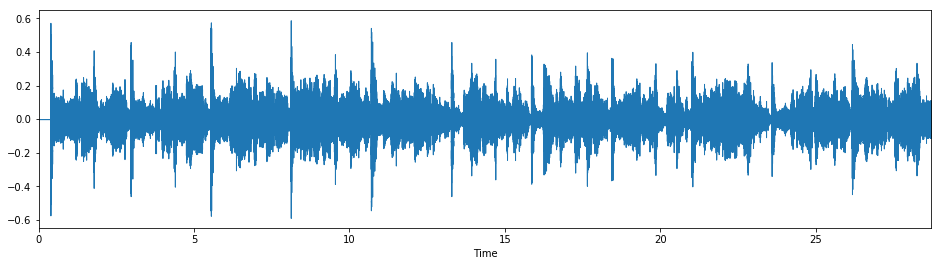Much better! With clicks:

In :
overlay_rhythm_onto_audio(target_rhythm, y_modified, measure_samples, sr)

Out: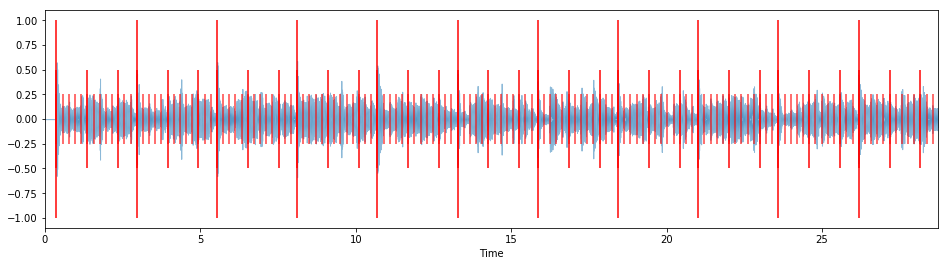As you can hear, the modified track's rhythm is in line with the clicks, and sounds noticeably different from the original song. This is a pretty good place to end up!

## Part 6 - Fibonacci stretch: implementation and examples¶

### 6.1 Implementation¶

Here's an end-to-end implementation of Fibonacci stretch. A lot of the default parameters have been set to the ones we've been using in this notebook, although of course you can pass in your own:

In :
def fibonacci_stretch_track(audio_filepath,
sr=44100,
original_rhythm=np.array([1,0,0,1,0,0,1,0], dtype="int"),
stretch_method="euclidean",
stretch_factor=1,
target_rhythm=None,
tempo=None,
beats_per_measure=4,
hop_length=1024,
overlay_clicks=False,
render_track=True):

# Extract rhythm features from audio
if tempo is None:
tempo = estimate_tempo(y, sr)
beat_times = calculate_beat_times(y, sr, tempo)
beat_samples = librosa.time_to_samples(beat_times, sr=sr)
measure_samples = calculate_measure_samples(y, beat_samples, beats_per_measure)

# Generate target rhythm
if target_rhythm is None:
target_rhythm = fibonacci_scale_rhythm(original_rhythm, stretch_factor)

# Modify the track
y_modified, measure_samples_modified = modify_track(y, measure_samples,
original_rhythm, target_rhythm,
stretch_method="euclidean")

# Render the modified track...
if render_track:
if overlay_clicks:
return overlay_rhythm_onto_audio(target_rhythm, y_modified, measure_samples_modified, sr)
else:
plt.figure(figsize=(16,4))
librosa.display.waveplot(y_modified, sr=sr)
return ipd.Audio(y_modified, rate=sr)
# ... or return modified track and measure samples
else:
return (y_modified, measure_samples_modified)


Now we can simply feed the function a path to an audio file (as well as any parameters we want to customize).

This is the exact method that's applied to the sneak peek at the final result up top. The only difference is that we use a 90-second excerpt rather than our original 30-second one:

In :
# "Human Nature" stretched by a factor of 1 using default parameters
fibonacci_stretch_track("data/humannature_90s.mp3",
stretch_factor=1,
tempo=93.0)

Out: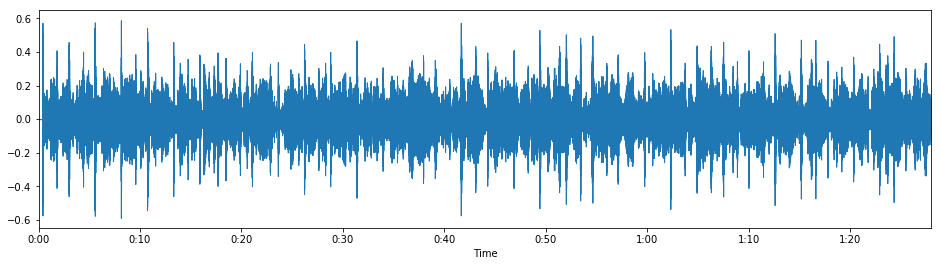And indeed we get the exact same result.

### 6.2 Examples: customizing stretch factors¶

Now that we have a function to easily stretch tracks, we can begin playing around with some of the parameters.

Here's the 30-second "Human Nature" excerpt again, only this time it's stretched by a factor of 2 instead of 1:

In :
# "Human Nature" stretched by a factor of 2
fibonacci_stretch_track("data/humannature_30s.mp3",
tempo=93.0,
stretch_factor=2,
overlay_clicks=True)

Out: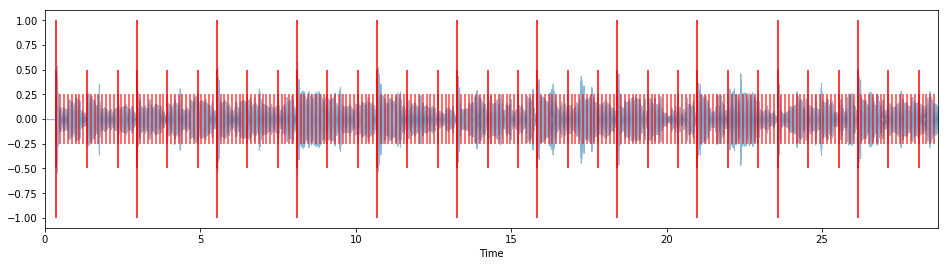As mentioned in part 2.2, we can contract rhythms as well using negative numbers as our stretch_factor. Let's try that with "Chan Chan" by the Buena Vista Social Club:

In :
# "Chan Chan" stretched by a factor of -1
fibonacci_stretch_track("data/chanchan_30s.mp3",
stretch_factor=-1,
tempo=78.5)

Out: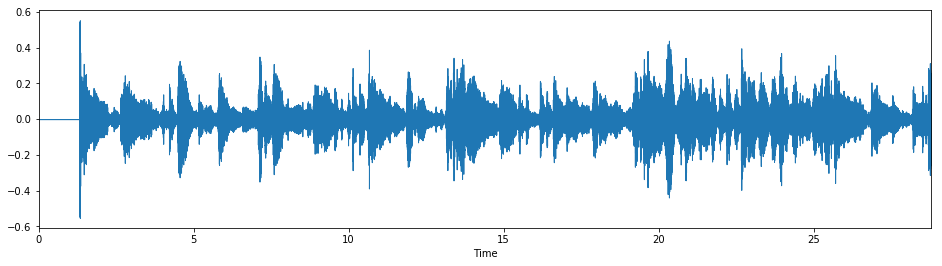(Note that although we do end up with a perceptible difference (the song now sounds like it's in 7/8), it should actually sound like it's in 5/8, since [1 0 0 1 0 0 1 0] is getting compressed to [1 0 1 0 1]. This is an implementation detail with the Euclidean stretch method that I need to fix.)

### 6.3 Examples: customizing original and target rhythms¶

In order to get musically meaningful results we generally want to supply parameters that make musical sense with our input audio (although it can certainly be interesting to try with parameters that don't!). One of the parameters that makes the most difference in results is the rhythm sequence used to represent each measure.

Here's Chance the Rapper's verse from DJ Khaled's "I'm the One", with a custom original_rhythm that matches the bassline of the song:

In :
# "I'm the One" stretched by a factor of 1
fibonacci_stretch_track("data/imtheone_cropped_chance_60s.mp3",
tempo=162,
original_rhythm=np.array([1,0,0,0,0,1,0,0]),
stretch_factor=1)

Out: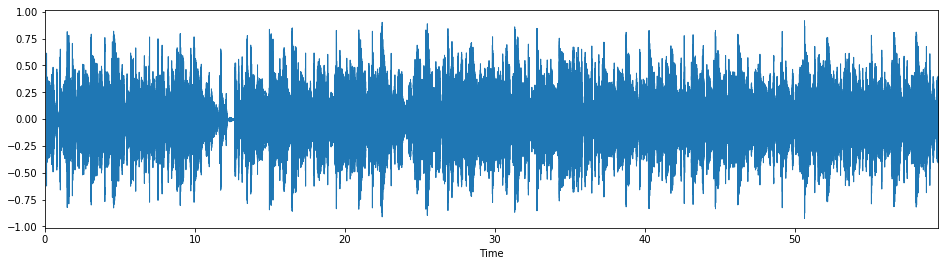We can define both a custom target rhythm as well. In addition, neither original_rhythm nor target_rhythm have to be Fibonacci rhythms for the stretch algorithm to work (although with this implementation they do both have to have the same number of pulses).

Let's try that out with the same verse, going from an original rhythm with 8 steps (i.e. in 4/4 meter) to a target rhythm with 10 steps (i.e. in 5/4 meter):

In :
# "I'm the One" in 5/4
fibonacci_stretch_track("data/imtheone_cropped_chance_60s.mp3",
tempo=162,
original_rhythm=np.array([1,0,0,0,0,1,0,0]),
target_rhythm=np.array([1,0,0,0,0,1,0,0,0,0]),
overlay_clicks=True)

Out: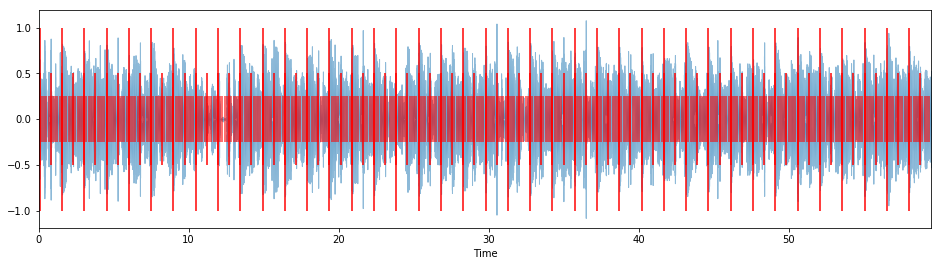As another example, we can give a swing feel to the first movement of Mozart's "Eine kleine Nachtmusik" (K. 525), as performed by A Far Cry:

In :
# "Eine kleine Nachtmusik" with a swing feel
fibonacci_stretch_track("data/einekleinenachtmusik_30s.mp3",
tempo=130,
original_rhythm=np.array([1,0,1,1]),
target_rhythm=np.array([1,0,0,1,0,1]))

Out: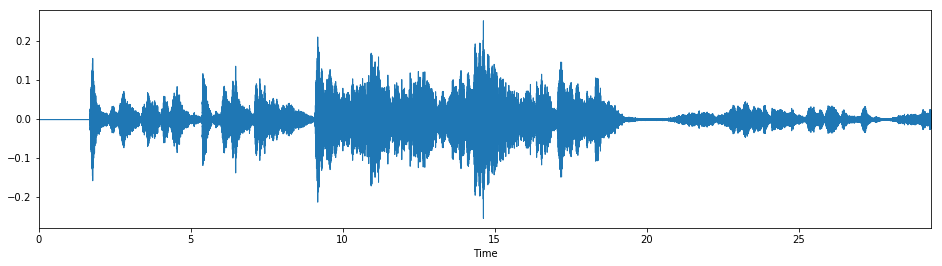It works pretty decently until around 0:09, at which point the assumption of a metronomically consistent tempo breaks down. (This is one of the biggest weaknesses with the current implementation, and is something I definitely hope to work on in the future.)

Let's also hear what "Chan Chan" sounds like in 5/4:

In :
# "Chan Chan" in 5/4
fibonacci_stretch_track("data/chanchan_30s.mp3",
tempo=78.5,
original_rhythm=np.array([1,0,0,1,0,0,0,0]),
target_rhythm=np.array([1,0,0,0,0,1,0,0,0,0])) # Also interesting to try with [1,0,1]

Out: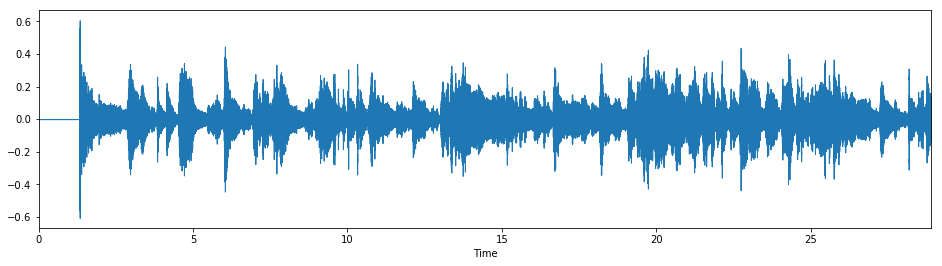### 6.4 Examples: customizing input beats per measure¶

We can also work with source audio in other meters. For example, Frank Ocean's "Pink + White" is in 6/8. Here I've stretched it into 4/4 using the rhythm of the bassline, but you can uncomment the other supplied parameters (or supply your own!) to hear how they sound as well:

In :
# "Pink + White" stretched by a factor of 1
fibonacci_stretch_track("data/pinkandwhite_30s.mp3",
beats_per_measure=6,
tempo=160,

# 6/8 to 4/4 using bassline rhythm
original_rhythm=np.array([1,1,1,1,0,0]),
target_rhythm=np.array([1,1,1,0,1,0,0,0]),

# 6/8 to 4/4 using half notes
# original_rhythm=np.array([1,0,0,1,0,0]),
# target_rhythm=np.array([1,0,0,0,1,0,0,0]),

# 6/8 to 10/8 (5/4) using Fibonacci stretch factor of 1
# original_rhythm=np.array([1,0,0,1,0,0]),
# stretch_factor=1,

overlay_clicks=True)

Out: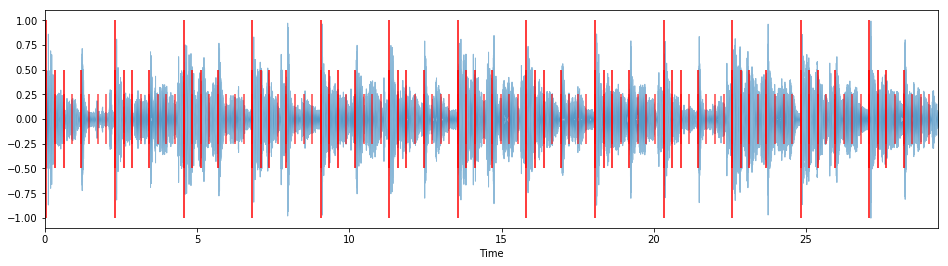## Part 7 - Final thoughts¶

This notebook started out as an answer to the question "What if we applied rhythmic expansion methods, based on the Fibonacci sequence, to actual audio tracks?"

It quickly grew into more and more, and we now have a working implementation of what I've dubbed Fibonacci stretch. Along the way I've come to a few conclusions that I'll go over:

### 7.1 Fibonacci stretch as a creative tool¶

I think there's certainly a case to be made for Fibonacci stretch as an interesting and useful means of musical transformation; it's rooted in mathematical processes that have been shown to produce interesting artistic output, and Part 6 shows that Fibonacci itself can produce musically interesting results.

However, it has its limits as well, the main one being that the Fibonacci sequence grows at an exponential rate (with the rate actually being equal to the golden ratio). This means that above a certain stretch_factor value, Fibonacci stretch starts to feel somewhat impractical. For example, stretching [1 0 0 1 0 0 1 0] by a factor of 6 gives us a target rhythm with 144 steps, which isn't something we can easily perceive when crammed into the space of our original 8 steps.

One solution to this imperceptibility is to allow for the length of the modified measure to change as well. For example, a 2-second measure with 8 steps (4 steps/second) could be stretched into a 24-second measure with 144 steps (6 steps/second). The longer time span might yield more interesting results, but it might also obscure the relationship between the modified track and the original track.

### 7.2 Rhythm perception¶

I gleaned a lot of insight from experimenting with different parameters. As shown in Part 2.2, the greater the stretch_factor, the closer we get to the golden ratio; with regards to rhythm perception, I found that this made the resulting rhythm sound more and more "natural", in an almost uncanny valley manner. This relates back to the limitations of Fibonacci stretch as a creative tool as well. Perhaps it would be worth examining the space of results that lie between stretch factors of 1 and 3, as those seem to be where the most musically interesting rhythmic shifts occur.

Similarly, plugging in different patterns for the original_rhythm and target_rhythm parameters yielded differing results, with some seeming more closely related to others. It's possible that there are some underlying rhythmic principles that more clearly explain how the relationship between the original and target rhythms affects how we perceive the stretched result.

In addition, the initially disappointing results of using naive time-stretch also indicate the importance of considering different perceptual levels of rhythm. We explored a solution using subdivision of pulses, but haven't dealt with perceptual levels of rhythm in a rigorous manner at all.

### 7.3 Implementation improvements¶

The current implementation of Fibonacci stretch works well enough, but it also leaves a lot to be desired.

One of the biggest issues is the handling of tempo. Firstly, the built-in tempo detection almost never gives us the correct value, which is why for the examples we've had to pass in tempo values manually. Looking into alternate tempo detection methods could mitigate this. More importantly, however, the current implementation doesn't allow for variable tempo, which is a real problem with tracks that weren't recorded to a metronome. Simply using a dynamic tempo estimate (which librosa.beat.tempo is capable of) would go a long way into improving the quality of the modified tracks.

This implementation of Fibonacci stretch also doesn't work too well when stretch_factor < 0. As with the "Chan Chan" example in Part 6.2, we don't get the results we expect. It might just be a quirk in the step length conversion in euclidean_stretch(), but it's also possible that an adidtional level of subdivision might be needed.

We might also want to explore using onset detection to improve the actual time-stretching process. Instead of choosing stretch regions purely based on where they fall in relation to the symbolic rhythm parameters, we could define regions in accordance with the sample indices of detected onsets as well, which could yield more natural sounding output.

### 7.4 Future directions¶

There are a ton of avenues to explore further, some of which we've touched upon. For example, some of the most interesting results came from stretching a track so that the performance was converted from one meter to another (e.g. 6/8 to 5/4). Sometimes this occurred as a happy coincidence of Fibonacci stretch, but most of the time we had to pass in custom original_rhythm and target_rhythm parameters. I think it would be worthwhile to explore a version of the stretch method that could convert meter A to meter B without explicitly defining the rhythm patterns.

On a related note, exploring the possibilities of Euclidean rhythms, outside of their relationship to Fibonacci rhythms, could be worthwhile as well. In addition, we could allow each measure to be stretched using different parameters by passing in a list of arguments, each corresponding to a measure or group of measures. This would allow for more flexibility and creative freedom when using Fibonacci stretch.

Finally, I could see this stretch method being used in the context of a digital audio workstation (DAW), with both the symbolic meter data for a project and the raw audio signal for a track being modified. If embedded directly into a DAW, this would open up possibilities for rapid experimentation of rhythm in a music production setting.

All in all, I hope this notebook presents an insightful exploration of how the Fibonacci sequence can be applied to sample-level audio manipulation of rhythmic relationships!